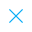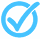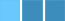Call Support +91-85588-96644

Keep me logged in
You can't leave Captcha Code empty
By submitting this form, you agree to the Terms & Privacy Policy.
ORTests given

OR# FLT - 4 Mathematics (X - 2018)

Topics Covered:TrianglesArithmetic ProgressionsCirclesHCF and LCM Using Prime FactorizationIntroduction to TrigonometryPair of Linear Equations in Two VariablesSimple Problems on Distance FormulaSurface Areas and VolumesTheoretical Concepts of ProbabilityCalculating Mean of Grouped Data

This pdf is currently unavailable.
Description:
Mathematics, Class - X, Triangle, Trigonometry, Polynomials, Quadratic Equation, Divisibility Rule
Keywords: